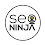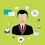## Saturday, July 2, 2011

### The R apply function – a tutorial with examples

Today I had one of those special moments that is uniquely associated with R. One of my colleagues was trying to solve what I term an 'Excel problem'. That is, one where the problem magically disappears once a programming language is employed. Put simply, the problem was to take a range, and randomly shift the elements of the list in order. For example, 12345 could become 34512 or 51234.

The list in question had forty-thousand elements, and this process needed to be repeated numerous times as part of a simulation. Try doing this in Excel and you will go insane: the shift function is doable but resource intensive. After ten minutes of waiting for your VBA script to run you will be begging for mercy or access to a supercomputer. However, in R the same can be achieved with the function:
```translate<-function(x){
if (length(x)!=1){
r<-sample(1:(length(x)),1)
x<-append(x[r:length(x)],x[1:r-1])
}
return(x)```
```}
```
My colleague ran this function against his results several thousand times and had the pleasure of seeing his results spit out in less than thirty seconds: problem solved. Ain't R grand.

More R magic courtesy of the apply function
The translate function above is not rocket science, but it does demonstrate how powerful a few lines of R can be. This is best exemplified by the incredible functionality offered by the apply function. However, I have noticed that this tool is often under-utilised by less experienced R users.

The usage from the R Documenation is as follows:
`apply(X, MARGIN, FUN, ...)`

where:
• X is an array or matrix;
• MARGIN is a variable that determines whether the function is applied over rows (MARGIN=1), columns (MARGIN=2), or both (MARGIN=c(1,2));
• FUN is the function to be applied.
In essence, the apply function allows us to make entry-by-entry changes to data frames and matrices. If MARGIN=1, the function accepts each row of X as a vector argument, and returns a vector of the results. Similarly, if MARGIN=2 the function acts on the  columns of X. Most impressively,  when MARGIN=c(1,2) the function is applied to every entry of X. As for the FUN argument, this can be anything from a standard R function, such as sum or mean, to a custom function like translate above.

An illustrative example
Consider the code below:
```# Create the matrix
m<-matrix(c(seq(from=-98,to=100,by=2)),nrow=10,ncol=10)

# Return the product of each of the rows
apply(m,1,prod)

# Return the sum of each of the columns
apply(m,2,sum)

# Return a new matrix whose entries are those of 'm' modulo 10
apply(m,c(1,2),function(x) x%%10) ```

In the last example, we apply a custom function to every entry of the matrix. Without this functionality, we would be at something of a disadvantage using R versus that old stalwart of the analyst: Excel. But with the apply function we can edit every entry of a data frame with a single line command. No autofilling, no wasted CPU cycles.

In the next edition of this blog, I will return to looking at R's plotting capabilities with a focus on the ggplot2 package. In the meantime, enjoy using the apply function and all it has to offer.

1.Andrej-Nikolai SpiessJuly 2, 2011 at 11:59 AM

You can do the mod call directly on the matrix

m%%10

This is also much faster than margin = c(1, 2):

system.time(for (i in 1:10000) apply(m,c(1,2),function(x) x%%10))

system.time(for (i in 1:10000) m%%10)

Cheers, Andrej

2.# Return the mean of each of the columns
apply(m,2,sum)

Isn´t here a typo?

3.Thanks for your comments. You are dead right, Andrej, the mod call can be done directly on the matrix. However, for the purposes of illustration I have used the apply function to demonstrate its application.

EDI, thanks for picking up that typo.

4.sample(x, length(x)) ?

5.I have to question your statements about the speed of excel to do this problem. I wrote the following function and ran it over 100 thousand times in less than a second.

Public Function Rearrange(X)
Dim pos As Integer

If Len(X) <= 1 Then
Rearrange = X
Else
pos = Int(Rnd() * Len(X)) + 1

Rearrange = Mid(X, pos, 1) & Rearrange(Mid(X, 1, pos - 1) & Mid(X, pos + 1))
End If

End Function

6.I agree that the speed problem is greatly overstated. But it doesn't even take VBA to do this in Excel.
Column A: the number(s) to rotate digits, formatted as text
Column B: =RANDBETWEEN(1, LEN(A1))
Column C: =CONCATENATE(RIGHT(A1,B1),LEFT(A1,LEN(A1)-B1))
Seems to satisfy the specification and runs PDQ

7.This comment has been removed by the author.

8.Excellent.....!!
If you are looking for Microsoft assistance for www office com/setup or Install and enter product key with Genuine Product Serial Key then you can visit our website or click on given link. Thanks
office.com/setup
office com setup
www office com/setup
www.office.com/setup